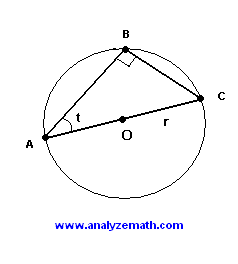# Maximum Area of Triangle - Optimization Problem with Solution

The first derivative is used to maximize the area of a triangle inscribed in a circle. An optimization problem with solution.

## Problem

In the picture below triangle ABC is inscribed inside a circle of center O and radius r. For a constant radius r of the circle, point B slides along the circle so that the area of ABC changes. Find the length of sides AB and CB so that the area of triangle ABC is maximum.Solution to Problem:

• Since the center O of the circle is on the side AC of the triangle, AC is a diameter of the circle and triangle ABC is a right triangle (Thales's theorem). Its right angle is at B and its hypotenuse AC has length equal to 2r.(see figure below)• The area S of the triangle is given by
S = (1 / 2) AB * CB
• Using angle t, AB and CB may be expressed as follows
AB = AC * cos t and CB = AC * sin t
• Substitute AC by AC * cos t and CB by AC * sin t in the above formula for the area, we obtain
S = (1/2) AC 2 cos t sin t
• We now use the trigonometric formula sin(2t) = 2 sin t cos t to express the area S as follows.
S = (1/4)AC 2 sin (2t)
• Since the radius r is constant, the length of the diameter AC is also constant, hence the area depends on angle t only as point B slides along the circle. To find t so that S is maximum, we need to find the first derivative and the stationary points.
dS / dt = (1/4)AC 2 2 cos (2t)
• We now equate dS / dt to zero to find stationary points and interval of increase and decrease.
(1/4)AC 2 2 cos (2t) = 0
• As point B slides along the circle, angle t changes fro 0 to 90 degrees. So the only solution to the above equation in the inteval (0 , 90) is
2 t = 90 or t = 45 degrees
• As t changes from 0 to 45 , 2t changes from 0 to 90 and dS / dt is positive on this interval. As t changes from 45 to 90, 2t chnages from 90 to 180 degrees and dS / dt is negative on this interval. t = 45 degrees is the location of a maximum value for the area S.
• When t = 45 degrees, the area of the inscribed right triangle is maximum. The length of sides AB and CB are given by
AB = AC * cos (45 degrees) = 2 r sqrt(2)
and CB = AC * sin (45 degrees) = 2 r sqrt(2)
• The area is maximum when t = 45 degrees which also means that the right triangle is isosceles.

More references on calculus problems